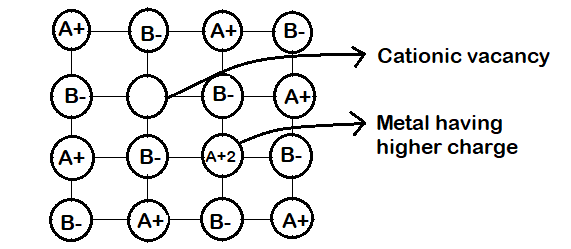QuestionAnswers

# Which of the following will have metal deficiency defects?A.NaClB.FeO C.KCl D.ZnO

Hint: Metal deficiency defects are caused due to missing cations. So, one of the nearest metal ions acquires an extra positive charge to maintain electrical neutrality and to do so the metal ion must be able to exhibit variable valency.-An example of such solids is FeO. The composition of FeO may range from $F{e_{0.93}}O$ to $F{e_{0.96}}O$. But usually it is found with a composition of $F{e_{0.95}}O$. In FeO crystals some $F{e^{ + 2}}$ ions are missing, so this loss of positive ions is made up by turning some $F{e^{ + 2}}$ ions into $F{e^{ + 3}}$ ions.